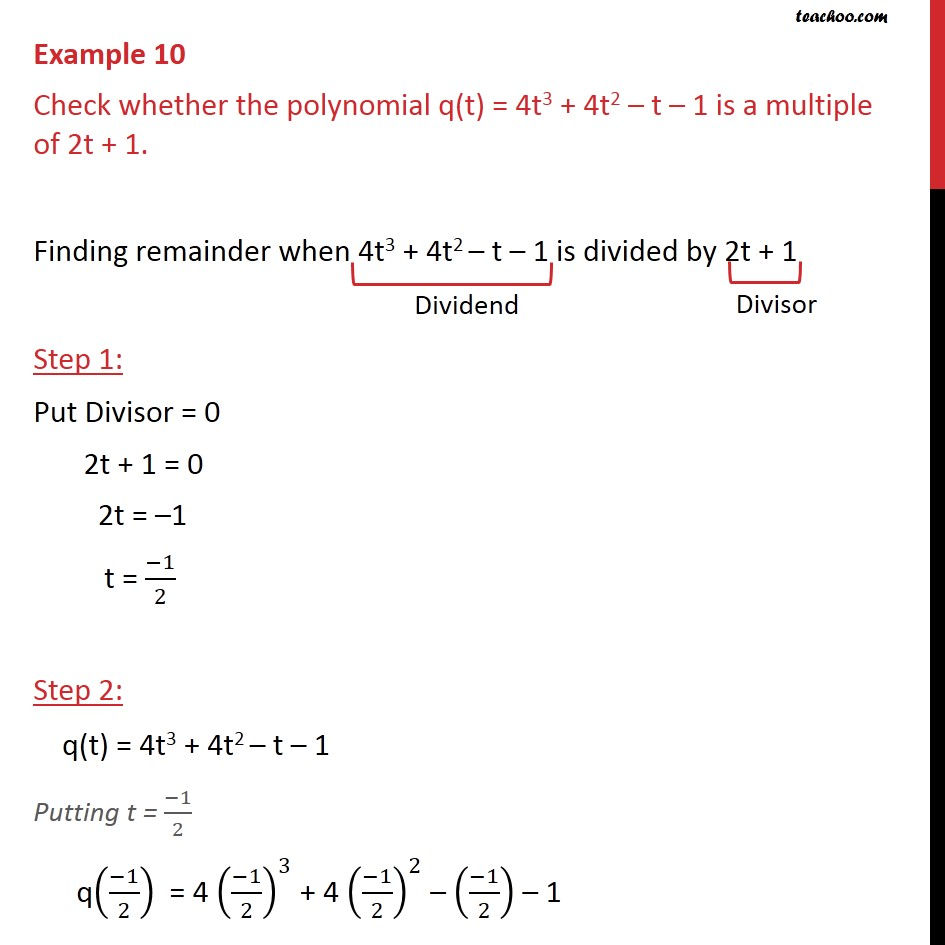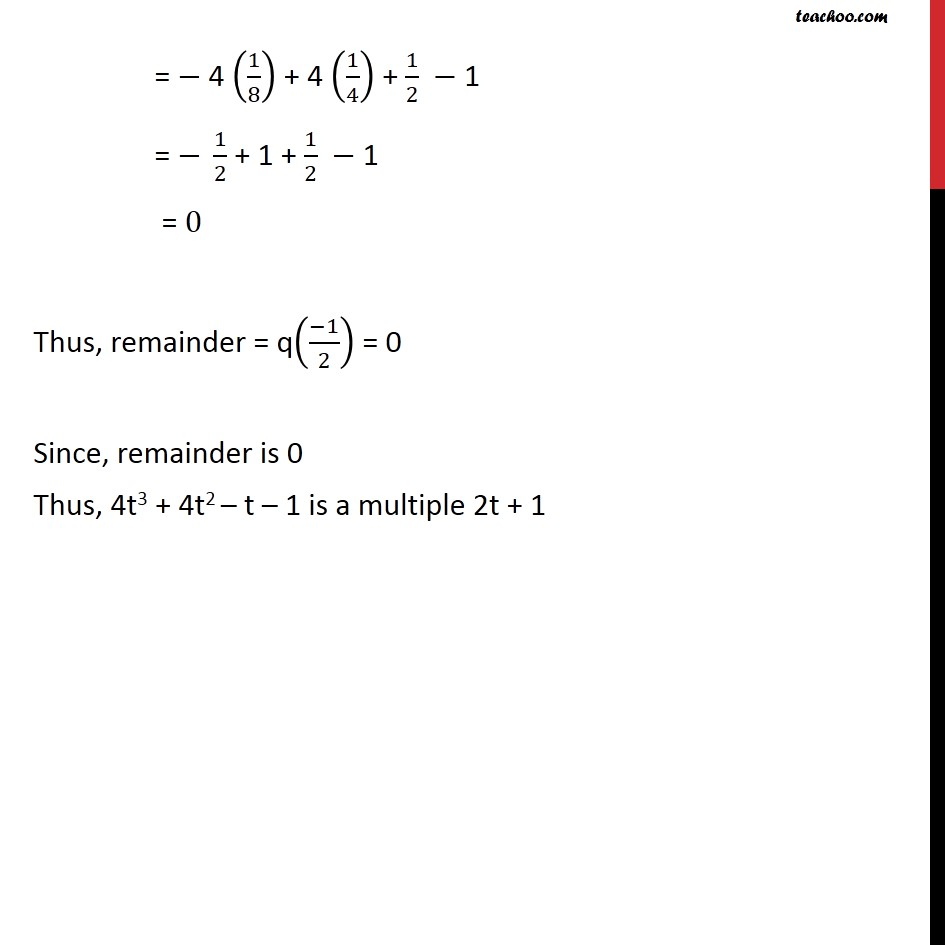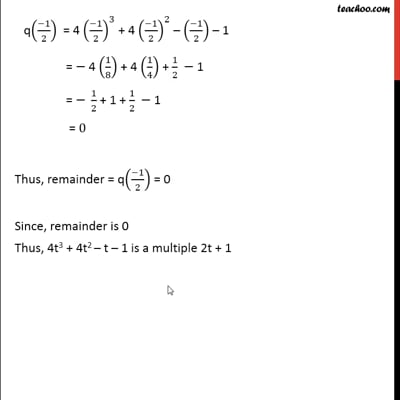Examples

Chapter 2 Class 9 Polynomials
Serial order wiseThis video is only available for Teachoo black users

Solve all your doubts with Teachoo Black (new monthly pack available now!)

### Transcript

Example 10 Check whether the polynomial q(t) = 4t3 + 4t2 – t – 1 is a multiple of 2t + 1. Finding remainder when 4t3 + 4t2 – t – 1 is divided by 2t + 1 Step 1: Put Divisor = 0 2t + 1 = 0 2t = –1 t = (−1)/2 Step 2: q(t) = 4t3 + 4t2 – t – 1 Putting t = (−1)/2 q((−1)/2) = 4 ((−1)/2)^3 + 4 ((−1)/2)^2 – ((−1)/2) – 1 = − 4 (1/8) + 4 (1/4) + 1/2 − 1 = − 1/2 + 1 + 1/2 − 1 = 0 Thus, remainder = q((−1)/2) = 0 Since, remainder is 0 Thus, 4t3 + 4t2 – t – 1 is a multiple 2t + 1